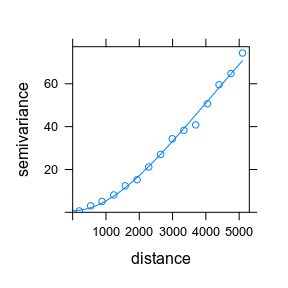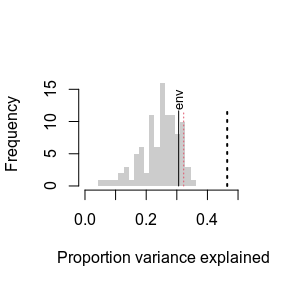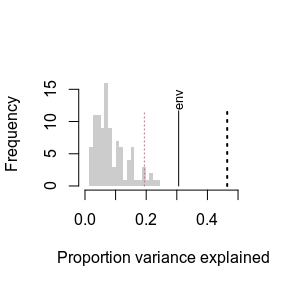# randomTF on spatially structured environments

#### 2023-03-09

Spatial autocorrelation can severely bias transfer function performance estimates. If can also bias reconstruction significance tests, but I suspect the bias is not as severe.

This vignette show how to use the autosim argument to randomTF() to use an autocorrelated simulated environmental variables, instead of the default uniformly distributed independent environmental variables, to make the reference distribution.

library(palaeoSig)
library(rioja)
library(sf)
library(gstat)
library(dplyr)
library(tibble)
library(tidyr)
library(purrr)
library(ggplot2)
set.seed(42) # for reproducibility 

## Data

We use the foraminifera dataset by Kucera et al. (2005). We use some of the samples to represent a core.

# load data
data(Atlantic)
meta <- c("Core", "Latitude", "Longitude", "summ50")
Atlantic <- as.data.frame(Atlantic) # prevents rowname warnings

# pseudocore as no fossil foram data in palaeoSig
fosn <- Atlantic |>
filter(between(summ50, 5, 10)) |>
slice_sample(n = 20)

# remaining samples as training set
Atlantic <- Atlantic |>
anti_join(fosn, by = "Core") |>
slice_sample(n = 300) # random subset to speed analysis up

Atlantic_meta <- Atlantic |>
select(one_of(meta)) # to keep rdist.earth happy
Atlantic <- Atlantic |> # species
select(-one_of(meta))

fos <- fosn |>
select(-one_of(meta))

## Variogram

We need to convert the meta data into an sf object for further calculation.

Atlantic_meta <- st_as_sf(
x = Atlantic_meta,
coords = c("Longitude", "Latitude"),
crs = 4326
)

Fitting the variogram is the hardest part. There are several types of variogram model available, e.g. exponential “Exp”, spherical “Sph”, gaussian “Gau” and Matérn “Mat”. These have different shapes. It is important to find one that fits the data well.

# Estimate the variogram model for the environmental variable of interest
ve <- variogram(summ50 ~ 1, data = Atlantic_meta)
vem <- fit.variogram(
object = ve,
model = vgm(40, "Mat", 5000, .1, kappa = 1.8)
)
plot(ve, vem)Figure 1: Semi-variogram model (Matérn class) fitted to the Atlantic summer sea temperature at 50 m depth.

vem
#>   model       psill    range kappa
#> 1   Nug   0.7358321    0.000   0.0
#> 2   Mat 185.3131517 3404.449   1.8

## Kriging

Now we can use gstat::krige to do Gaussian unconditional simulation and make simulated environmental fields with the same spatial structure as the observed variable. This step is quite slow with large datasets.

# Simulating environmental variables
sim <- krige(sim ~ 1,
locations = Atlantic_meta,
dummy = TRUE,
nsim = 100,
beta = mean(Atlantic_meta$"summ50"), model = vem, newdata = Atlantic_meta ) #> [using unconditional Gaussian simulation] # convert sf back to a regular data.frame sim <- sim |> st_drop_geometry() Now we can run randomTF using the simulated environmental variables. rtf_auto <- randomTF( spp = Atlantic, env = Atlantic_meta$summ50,
fos = fos,
autosim = sim,
fun = MAT,
col = "MAT.wm"
)
#> Warning in Merge(object$y, newdata, split = TRUE): Some row names were changed #> to avoid duplicates. #> Warning in Merge(object$y, newdata, split = TRUE): Some row names were changed
#> to avoid duplicates.

plot(rtf_auto)Figure 2. Result of randomTF with an autocorrelated null model.

rtf_ind <- randomTF(
spp = Atlantic,
env = Atlantic_meta$summ50, fos = fos, fun = MAT, col = "MAT.wm" ) #> Warning in Merge(object$y, newdata, split = TRUE): Some row names were changed
#> to avoid duplicates.

#> Warning in Merge(object\$y, newdata, split = TRUE): Some row names were changed
#> to avoid duplicates.

plot(rtf_ind)Figure 3. Result of randomTF with a spatially independent null model.